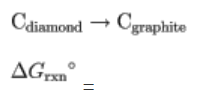# Problem: What is the standard Gibbs free energy for the transformation of diamond to graphite at 298 K?

###### FREE Expert Solution
94% (238 ratings)
###### FREE Expert Solution

The first thing we need for this problem are the Gibbs Free Energy of formation values:

C (graphite) = 0 kJ/mol

C (diamond) = 29 kJ/mol

The equation to find ΔGrxn

94% (238 ratings)###### Problem Details

What is the standard Gibbs free energy for the transformation of diamond to graphite at 298 K?